View Course Path

To implement a logic, we use logic circuits. There are two types of logic circuits – combinational logic circuits and sequential logic circuits. Combinational logic circuits are time-independent circuits that deploy boolean logic to achieve output. This output depends on the current input and nothing else. Arithmetic logic is necessary for any digital system, as we have seen earlier.

In this post, we will take a look at the different variants of an adder and a subtractor. These particular circuits form an integral part of modern ICs where they are found in the Arithmetic Logic Units (ALUs) and the Digital Signal Processing (DSP) units.

Contents

## How to design a Half Adder circuit?

A half adder is an arithmetic combinational logic circuit that adds two 1-bit inputs to give the sum and the carry generated as the output.

### Why is it called a half adder?

The half adder circuit adds two single bits and ignores any carry if generated. Since any addition where a carry is present isn’t complete without adding the carry, the operation is not complete. Hence the circuit is known as a half-adder. Let’s write the truth table using general boolean logic for addition

0 + 0 = 0

0 + 1 = 1

1 + 0 = 1

1 + 1 = 0, carry 1

### Truth table for a half adder

 A B SUM CARRY 0 0 0 0 0 1 1 0 1 0 1 0 1 1 0 1

From the above table, SUM is one when A=0 And B=1 Or (not and because A and B can have only one value at a time) when A=1 And B=0. So we can say that

SUM = A’B + AB’

which is equal to

SUM =${ A\oplus B }$

Note: Understand this shortcut for deriving the equation for an output by just looking at the truth table. This is quite handy for small truth tables, and you don’t need to draw K-maps for each of them.

Similarly, for the CARRY output, it is 1 only when A=1 and B=1. Hence,

CARRY = AB

From the equations above, it is clear that the circuit consists of an EXOR gate and an AND gate. Designing based on the equations, we get the following logic circuit for the half adder.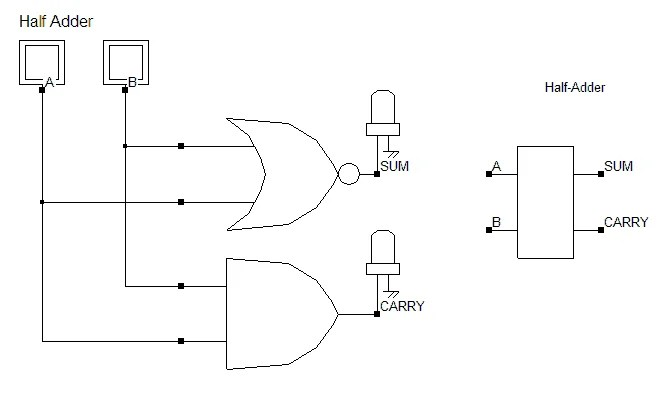## How to design a Full Adder circuit?

The only difference between a full adder and a half adder is that in a full adder, we also consider the carry input. So we have three inputs instead of two. Let’s plot the truth table using three inputs and general binary addition rules.

### Truth table for a full adder

 A B Y SUM CARRY 0 0 0 0 0 0 0 1 1 0 0 1 0 1 0 0 1 1 0 1 1 0 0 1 0 1 0 1 0 1 1 1 0 0 1 1 1 1 1 1

Solving for SUM output using K-maps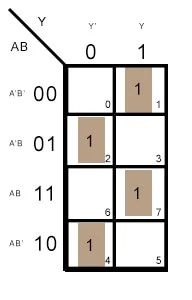SUM = A’B’Y + A’BY’ + ABY + AB’Y’ = Y(A’B’+AB) + Y'(A’B+AB’) = YX’+Y’X =${ Y\oplus X }$${ A\oplus B\oplus Y }$

Where X is the equation for EXOR and X’ is the equation for EXNOR.

Similarly for CARRY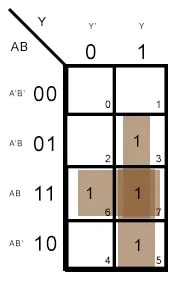CARRY = AB + BY + AY = Y(A+B) + AB

From the two equations of SUM and CARRY, we can design the following combinational logic circuit for a full adder.Compare the equations for half adder and full adder. The equation for SUM requires just an additional input EXORed with the half adder output. So we add the Y input and the output of the half adder to an EXOR gate. Similarly, for the carry output of the half adder, we need to add Y(A+B) in an OR configuration.## Half Subtractor

Quite similar to the half adder, a half subtractor subtracts two 1-bit binary numbers to give two outputs, difference and borrow. Since it neglects any borrow inputs and essentially performs half the function of a subtractor, it is known as the half subtractor. Let’s write the truth table based on this information and general binary subtraction rules.

0 – 0 = 0

0 – 1 = 1, borrow 1

1 – 0 = 1

1 – 1 = 0

### Truth table for a half subtractor

 A B DIFFERENCE BORROW 0 0 0 0 0 1 1 1 1 0 1 0 1 1 0 0

DIFFERENCE is high when A=0 and B=1 or when A=1 and B=0. Hence

DIFFERENCE= A’B + AB’ =$A \oplus B$

BORROW is high when A=0 and B=1. Hence

BORROW = A’B

From the equations for DIFFERENCE and BORROW## Full Subtractor

A full subtractor accounts for the borrow that a half subtractor neglects. Hence it has three inputs and two outputs. We will write the truth table for the full subtractor based on this information.

### Truth table for a full subtractor

 A B D DIFFERENCE BORROW 0 0 0 0 0 0 0 1 1 1 0 1 0 1 1 0 1 1 0 1 1 0 0 1 0 1 0 1 0 0 1 1 0 0 0 1 1 1 1 1

Solving for DIFFERENCE using Kmaps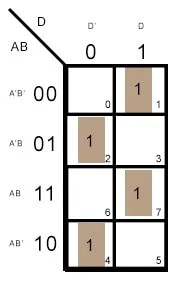This is similar to the Kmap for SUM for the full adder. The equation for DIFFERENCE is thus

DIFFERENCE =${ A\oplus B\oplus Y }$

Deriving the equation for BORROW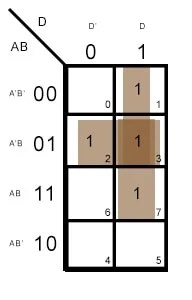BORROW = A’D + BD + A’B = A'(B+D) + BD

The circuit for the equations for DIFFERENCE and BORROW is as follows

## Full Subtractor using Half subtractor

Comparing the equations for a half subtractor and a full subtractor, the DIFFERENCE output needs an additional input D, EXORed with the output of DIFFERENCE from the half subtractor. And the BORROW output just needs two additional inputs DA’ and DB.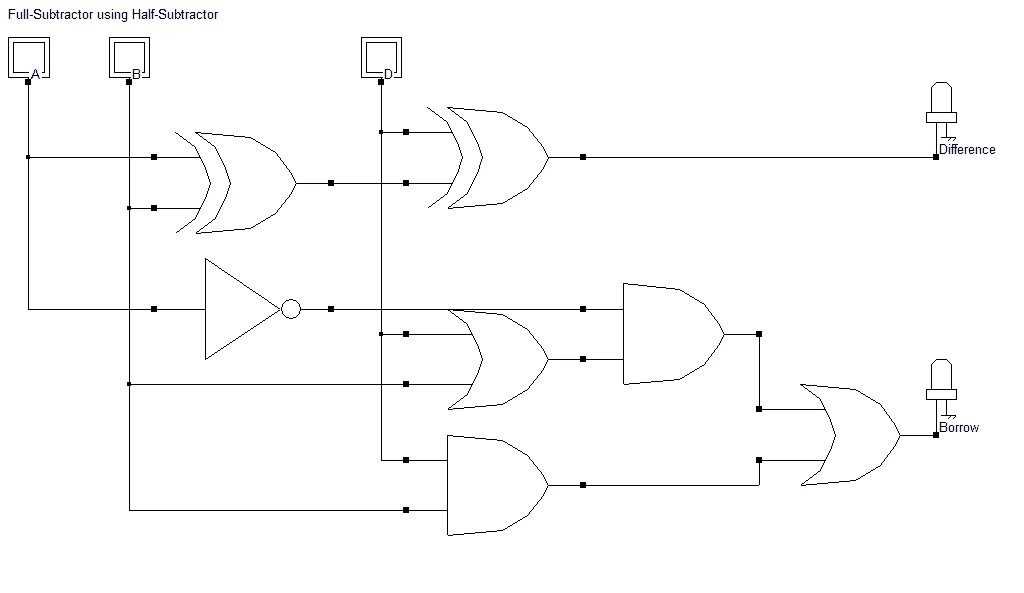Note: We will use all of the equations above when we code these combinational circuits in our VHDL and Verilog course.

## 4 thoughts on “Half Adder, Full Adder, Half Subtractor & Full Subtractor”

1.Gabriel Dalposso says:

I think making the difference in the full-subtractor just xor everything doesn’t consider the case where every input is 1…

1.Umair Hussaini says:

Why not? It totally does. An EXOR gate will give a high output when all the three inputs are one. That satisfies the truth table condition of the full subtractor.

2.vikas says:

nice computer concept full subtractor

1.Umair Hussaini says: